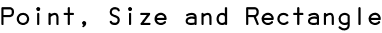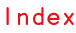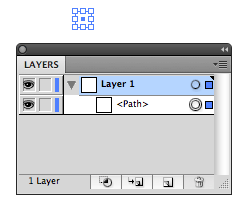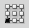RSS

In Scriptographer, basic types such as Point, Size and Rectangle are objects that describe geometric attributes of graphical items. They are an abstract representation of geometric values such as location and dimension, but do not directly represent a graphical item within the document.

Graphical items in the Illustrator document are items that appear in the layer list and visually in the document. An analogy can be drawn to 'physical' items in the real world. To describe their location and dimension, Scriptographer deploys different basic types, which are merely containers for numeric values that describe their geometric qualities.

This means that when we create a Point object in code, we are in fact only creating a description of a location in the document, but we are not creating a path item that contains this point as a segment:

```var myPoint = new Point(10, 20);
console.log(myPoint); // { x: 10, y: 20 }
```

In order to create a path item that contains this point as a segment, we would need to explicitly use the new Path() constructor to create a path and add the point as a first segment to it. The Working with Path Items tutorial describes paths and segments in more detail.

```var myPath = new Path();
```

Running this script now produces a 'physical' path item in the document with one segment at the location of myPoint:Again, the 'physically' appearing segment of myPath and the point called myPoint are not the same. myPoint is simply describing the coordinates that were used to produce the first segment of myPath. Modifying myPoint would not change that segment after it was created.

## Point

The Point object describes a two dimensional location. It has two properties x and y, representing the x and y coordinate of the location.

Point objects can be created either by directly providing the coordinates for x and y or by omitting them, in which case they are initialized with 0. The x and y coordinate properties can be accessed and changed separately too.

Here we are creating a new point without providing values for x and y, and then change the values after. The console.log() function is used to console.log the resulting values to the console.

```var myPoint = new Point();
console.log(myPoint); // { x: 0, y: 0 }

// Now let's change the x coordinate to 10...
myPoint.x = 10;

// ...and the y coordinate to x + 10
myPoint.y = myPoint.x + 10;
console.log(myPoint); // { x: 10, y: 20 }
```
Did you know?

The console.log() function sends text to the Scriptographer console and is very useful to debug scripts. Read more about console.log() in the Working with the Console tutorial.

Here we are creating a new point with defined coordinates, which are then modified.

```var myPoint = new Point(20, 40);
console.log(myPoint); // { x: 20, y: 40 }

// Now we are doubling the x coordinate by multiplying it with 2.
myPoint.x = myPoint.x * 2;
console.log(myPoint); // { x: 40, y: 40 }
```

Another way of creating a point object is by passing its constructor an existing point, of which the new point the becomes a copy. Changing the new point will not modify the original point:

```var firstPoint = new Point(20, 40);
var secondPoint = new Point(firstPoint);
console.log(secondPoint); // { x: 20, y: 40 }

secondPoint.y = 20;
console.log(secondPoint); // { x: 20, y: 20 }

// Note that firstPoint has not changed:
console.log(firstPoint); // { x: 20, y: 40 }

```

This is not the same as a simple variable reference, which does not make a copy:

```var firstPoint = new Point(20, 40);
var secondPoint = firstPoint;
secondPoint.y = 20;
console.log(secondPoint); // { x: 20, y: 20 }

// Note that firstPoint has changed as well:
console.log(firstPoint); // { x: 20, y: 20 }
```

All basic types in Scriptographer offer such a copying constructor. An easier way of making copies of objects in order to avoid modifying variables references is by calling the clone() function on any object:

```var firstPoint = new Point(20, 40);
var secondPoint = firstPoint.clone();
```

The Point object offers an alternative numeric way of describing the location, in opposition to the cartesian coordinate x, y: The properties angle and length, which describe the location in the polar coordinate system, defined by the distance (length) and angle to the coordinate sytstem's origin.

## Size

The Size object describes abstract dimensions in two dimensional space. It has two properties width and height, representing the width and height values of the size.

Just like with Point objects, Size objects can be created either by directly providing the values for width and height or by omitting them, in which case they are initialized with 0. The width and height properties can be accessed and changed separately too. As an example, the same steps from the Point object are executed with a Size object here. Anything that was said about the Point object applies to the Size object too, the only difference being the different property names.

```var mySize = new Size();
console.log(mySize); // { width: 0, height: 0 }

mySize.width = 10;
mySize.height = mySize.width + 10;
console.log(mySize); // { width: 10, height: 20 }
```
```var mySize = new Size(20, 40);
console.log(mySize); // { width: 20, height: 40 }

mySize.width = mySize.width * 2;
console.log(mySize); // { width: 40, height: 40 }
```

## Rectangle

The Rectangle object can be described as the combination of a Point object and a Size object, describing both a two dimensional location and a size. Therefore it posseses the four properties x, y, width and height. The properties x and y describe the coordinates of the top left point of the rectangle, width and height describe its dimensions. Additionally, it also defines the point and size properties, giving access to these values in form of Point and Size objects.

Rectangle objects can be created in a multitude of ways. One possibility is to pass a Point and a Size object to the new Rectangle(point, size) constructor:

```var topLeft = new Point(10, 20);
var rectSize = new Size(200, 100);
var rect = new Rectangle(topLeft, rectSize);
console.log(rect); // { x: 10, y: 20, width: 200, height: 100 }
console.log(rect.point); // { x: 10, y: 20 }
console.log(rect.size); // { width: 200, height: 100 }
```

The same Rectangle object can be created using the new Rectangle(x, y, width, height) constructor:

```var rect = new Rectangle(10, 20, 200, 100);
console.log(rect); // { x: 10, y: 20, width: 200, height: 100 }
```

A third possibility is to use the new Rectangle(point1, point2) constructor, which receives two corner points of the Rectangle object. These do not necessarily need to be the top left and bottom right corners, the constructor figures out how to fit a rectangle between them:

```var bottomLeft = new Point(10, 120);
var topRight = new Point(210, 20);
var rect = new Rectangle(bottomLeft, topRight);
console.log(rect); // { x: 10, y: 20, width: 200, height: 100 }
```

Newly created basic types do not necessarily need to be put into named variables every time. We could also nest constructor calls and pass them directly to the rectangle constructor.

```var rect = new Rectangle(new Point(10, 120),
new Point(210, 20));
```

## Rectangle Properties

The Rectangle object is a bit more complex than Point and Size objects and exposes a series of additional center and corner point objects: center, topLeft, topRight, bottomLeft, bottomRight, leftCenter, topCenter, rightCenter and bottomCenter. These correspond to the graphical options in the Transform Palette's Reference Point chooser:All these values can be changed after a rectangle is created, offering an easy ways to specify the geometric qualities of rectangles:

```// We start by creating a rectangle of dimension and
// location set to 0
var rect = new Rectangle();
console.log(rect); // { x: 0, y: 0, width: 0, height: 0 }

// Now we can for example define its size...
rect.size = new Size(100, 200);

// and its center
rect.center = new Point(100, 100);
console.log(rect); // { x: 50, y: 0, width: 100, height: 200 }
```

As an alternative numeric way to define the rectangle's dimensions, the Rectangle object also exposes the following properties, in opposition to x, y, width and height: left, top, right and bottom:

```var rect = new Rectangle();
rect.left = 100;
rect.right = 200;
rect.bottom = 400;
rect.top = 200;
console.log(rect); // { x: 100, y: 200, width: 100, height: 200 }
```